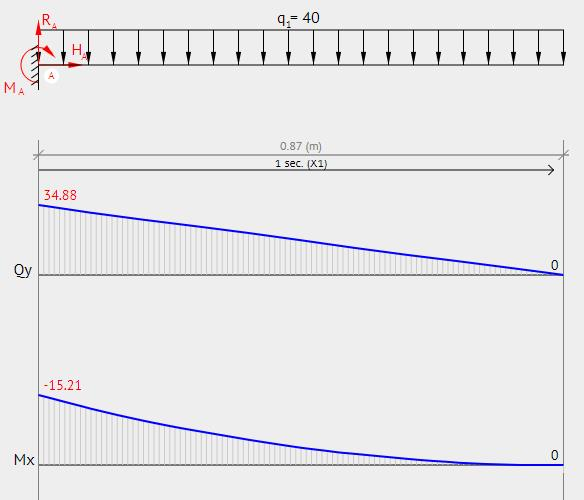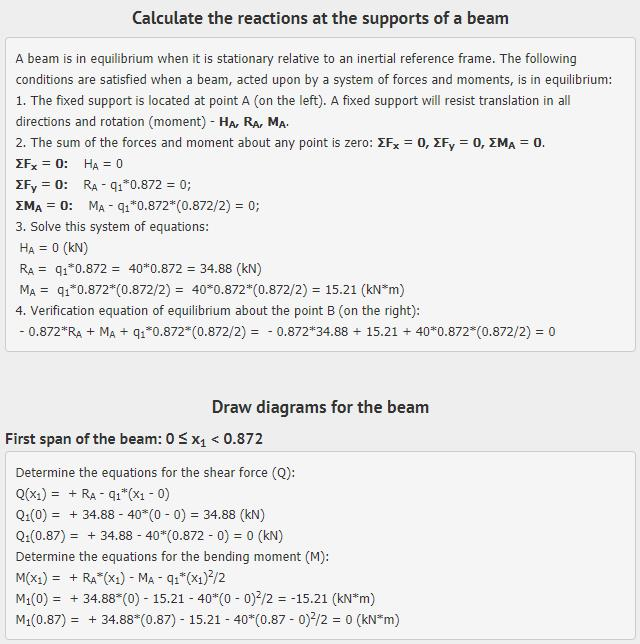peteroznewman
Subscriber

@Sandeep, the zip file attachment on this post has a paper with the definitions for Moment and Rotation.

Hello Fabricio,

From the paper, M = PL is equation (4), which is for a point load P at a distance L from the column.

You have used a distributed load, q = 40 kN/m for a length, L =  0.872 for the symmetric half model.

You calculate the beam shear and moment diagrams for your problem.  I used an online calculator.So when q = 40 kN/m,  M = 15.21 kNm

Regards,

Peter